# image dataset from directory in Tensorflow

In this post we will create tensorflow dataset(tf.data.Dataset) from MNIST image dataset using image_dataset_from_directory function

Here are the steps that we will follow for creating the MNIST tensorflow dataset to train the model:

• Setup Google colab and visualize the sample MNIST csv file
• Build a MNIST image dataset from the raw MNIST csv sample file in google colab
• Seggregate the images in image dataset into sub-directories corresponding to each class label i.e. digit 0-9
• Use image_dataset_from_directory function to split the dataset into train and validation set

Let’s get started

## Setup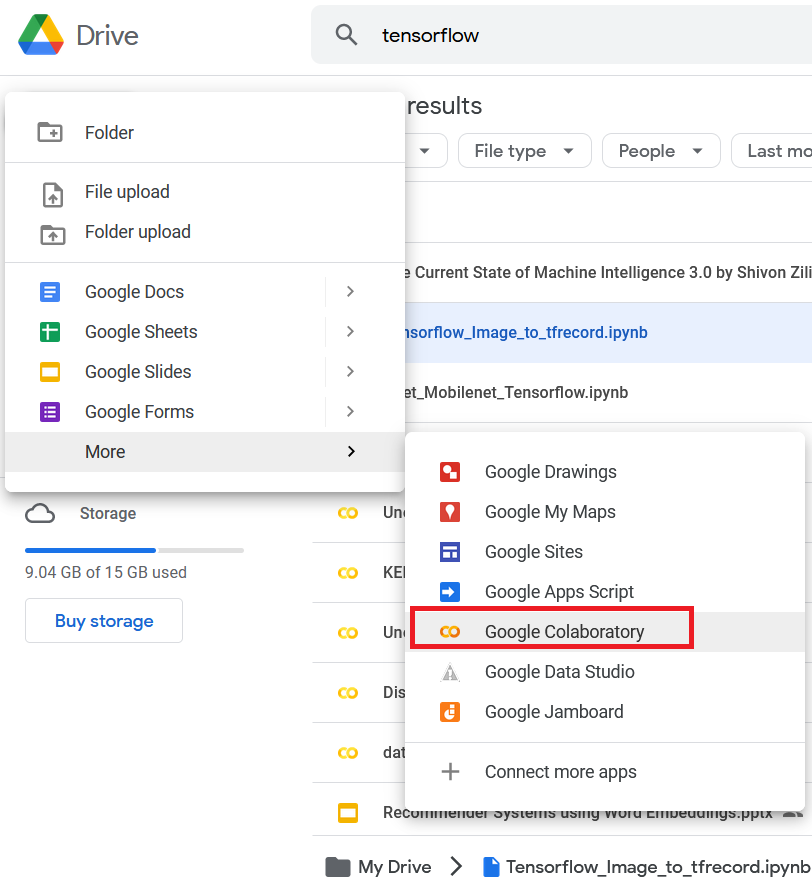Go to files tab and there you will find a default folder sample_data and mnist_train_small.csv file in it, this is our dataset file with 20,000 rows and 785 columns, the first column is the label and remaining 784 columns are pixel values of a 28x28 image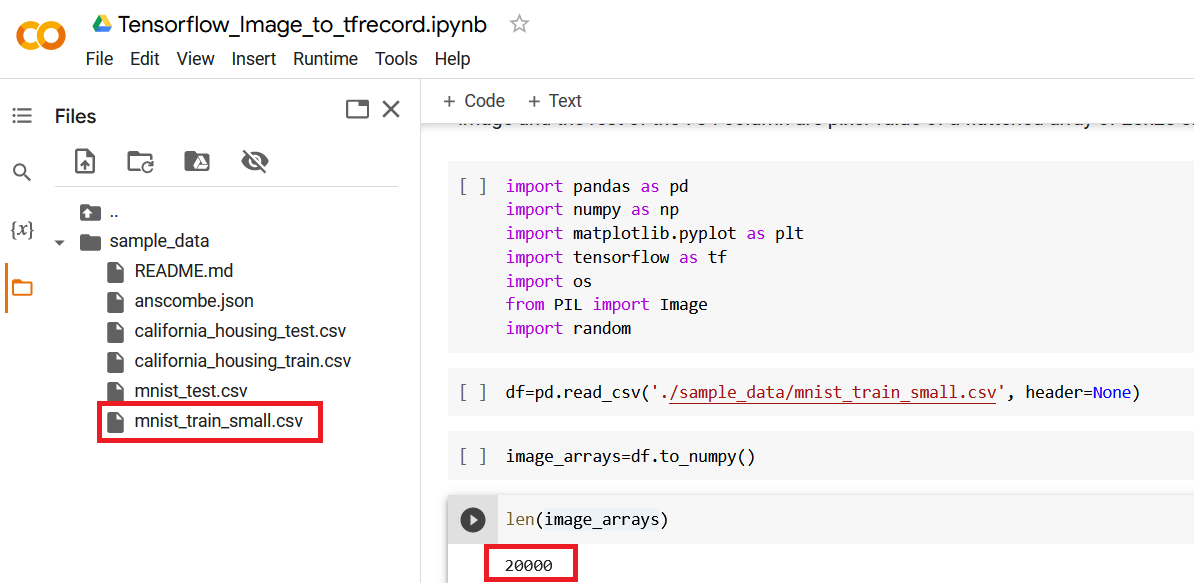## Build a MNIST image dataset

The raw dataset is a csv file,with 20,000 rows and 785 column. Each row represent an image with 784 columns that represents pixel value of a flattened 28x28 image array.

We will convert this csv dataset into an Image dataset with 20000 images(jpeg).

First we will read the csv file(mnist_train_small.csv) using pandas and load all the data in a pandas dataframe. After that convert the dataframe to a Numpy ndarray using to_numpy() function.

This function will yield a numpy.ndarray of size 20000x785, where the first column is the label of the image and the rest of the 784 column are pixel value of a flattened array of 28x28 size

``````import pandas as pd
import numpy as np
import matplotlib.pyplot as plt
import tensorflow as tf
import os
from PIL import Image
import random
import shutil
``````
``````df=pd.read_csv('./sample_data/mnist_train_small.csv', header=None)
``````
``````image_arrays=df.to_numpy()
len(image_arrays)
``````
``````Out:
20000
``````

Next, we need to store each image array as JPEG file with it’s label as file name in images folder.

Let’s create an images folder if does not exists already

``````#create images folder if not exists
if os.path.exists("images"):
shutil.rmtree("images")

os.makedirs("images")
``````

Now loop over the numpy ndarray(image_arrays) and save it as a jpeg file inside the images folder. The filename is a random 12 digit number followed by label of the image.

``````for img in image_arrays:
filename = os.path.join('images', str(random.randint(100000000000,999999999999))+f'_{img}.jpeg')
Image.fromarray(np.uint8(np.reshape(img[1:], (28,28)))).save(filename)
``````

Let’s check the count of images in folder

``````images=os.listdir(path)
len(images)

Out:
20000
``````

Now lets load and visualize one of the image. This ensures the images are stored successfully

``````images=os.listdir('images')
n=62
print(images[n])
plt.imshow(im)
``````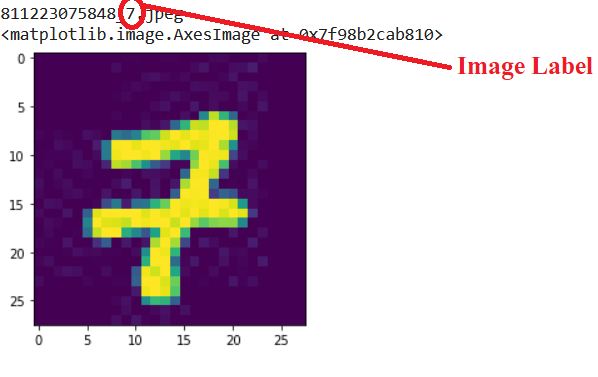## Create sub-directories for labels and move corresponding images in it

First we will create a function to get the label of each image from its filename

``````def get_label(filenm):
return filenm.split('.jpeg').split('_')
``````

Next, we will loop over the 20K images in the directory “images” and move each image under a sub-directory corresponding to the class it belongs. so we need to create 10 sub-directories for each digit labels from 0-9

``````path = 'images'
for image in images:
if not os.path.exists(os.path.join(path,get_label(image))):
os.makedirs(os.path.join(path,get_label(image)))
shutil.move(os.path.join(path,image),os.path.join(path, get_label(image),image))
``````

This is how the images directory will look like once all the images are moved under their corresponding class sub-directory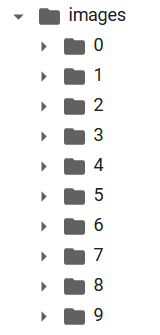## What is image_dataset_from_directory?

image_dataset_from_directory generates a a tf.data.Dataset from image files in a directory that yield batches of images from the sub-directories or class directory within the main directory and labels that is the sub-directory name

``````tf.keras.preprocessing.image_dataset_from_directory(
directory,
labels="inferred",
label_mode="int",
class_names=None,
color_mode="rgb",
batch_size=32,
image_size=(256, 256),
shuffle=True,
seed=None,
validation_split=None,
subset=None,
interpolation="bilinear",
crop_to_aspect_ratio=False,
**kwargs
)
``````

Arguments:

directory: Image Data path. Ensure it contains sub-directories of Image class if labels is set to “inferred”

labels: default is inferred (labels are generated from sub-directories of Image classes or a list/tuple of integer labels of same size as number of images in the directory label

label_mode: int - if labels are integers sparse_categorical_crossentropy loss categorical - labels are categorical for categorical_crossentropy loss binary - labels are either 0 or 1 for binary_crossentropy

class_names: Only if “labels” is “inferred” and is used for the order of classes otherwise it’s sorted in alphanumeric order

color_mode: image channels. default is ‘rgb’, if grayscale then it’s 1

batch_size: Image batch size, default is 32

image_size: default is (256,256)

shuffle: data shuffle, Boolean, default is True

validation_split: percent of data reserve for validation

subset: “training” for training data and “validation” for test data. Only used if validation_split is set.

Interpolation: default is bilinear and used when resizing image

crop_to_aspect_ratio: True or False, If set to true then resize image without aspect ratio distortion

Returns

A tf.data.Dataset object

## Use image_dataset_from_directory function to split the dataset into train and validation

Let’s define the batch size and required image height and width

``````batch_size = 32
img_height = 180
img_width = 180
``````

Let’s use 80% of the images for training, and 20% for validation.

we will create the train dataset and set the parameter subset to “validation” and validation split as 0.2

``````train_ds = tf.keras.utils.image_dataset_from_directory(
path,
validation_split=0.2,
subset="training",
seed=123,
image_size=(img_height, img_width),
batch_size=batch_size)
``````

Out:

``````Found 20000 files belonging to 10 classes.
Using 16000 files for training.
``````

Next we will create the validation dataset using image_dataset_from_directory function and set the parameter subset to “validation” and validation split as 0.2

``````val_ds = tf.keras.utils.image_dataset_from_directory(
path,
validation_split=0.2,
subset="validation",
seed=123,
image_size=(img_height, img_width),
batch_size=batch_size)
``````

Out:

``````Found 20000 files belonging to 10 classes.
Using 4000 files for validation.
``````

You can find the class names in the class_names attribute on these datasets. These correspond to the directory names in alphabetical order.

``````class_names = train_ds.class_names
print(class_names)
``````

Out:

``````['0', '1', '2', '3', '4', '5', '6', '7', '8', '9']
``````

Let’s visualize the data from the training dataset

Here are the first nine images from train_ds

``````import matplotlib.pyplot as plt

plt.figure(figsize=(10, 10))
for images, labels in train_ds.take(1):
for i in range(9):
ax = plt.subplot(3, 3, i + 1)
plt.imshow(images[i].numpy().astype("uint8"))
plt.title(class_names[labels[i]])
plt.axis("off")
``````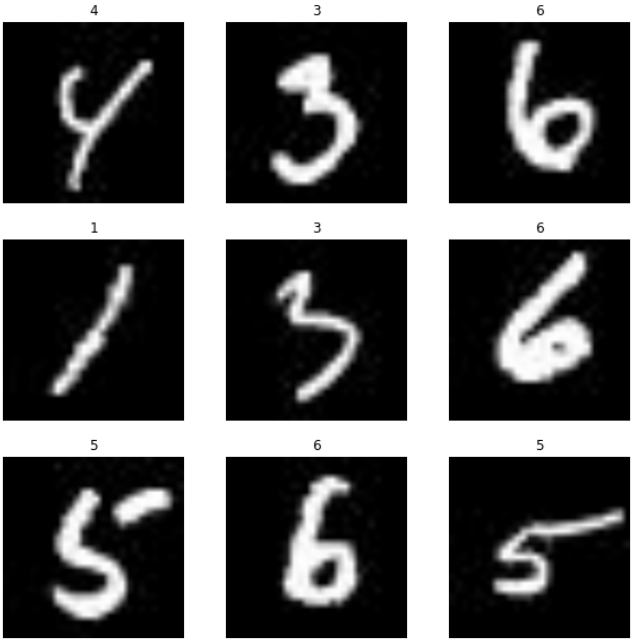## Get batches of Images and Labels from dataset

You can train a model using these datasets by passing them to Model.fit function. you can also manually iterate over the dataset and retrieve batches of images:

``````for image_batch, labels_batch in train_ds:
print(image_batch.shape)
print(labels_batch)
break
``````

out

``````(32, 180, 180, 3)
tf.Tensor([7 7 0 6 0 7 1 8 3 9 9 0 7 5 2 7 9 1 8 0 1 9 4 9 4 4 6 1 7 6 8 2], shape=(32,), dtype=int32)
``````

The image_batch is a tensor of the shape (32, 180, 180, 3). This is a batch of 32 images of shape 180x180x3 (the last dimension RGB channel).

The label_batch is a tensor of the shape (32,), these are corresponding labels to the 32 images.

Tags:

Categories:

Updated: